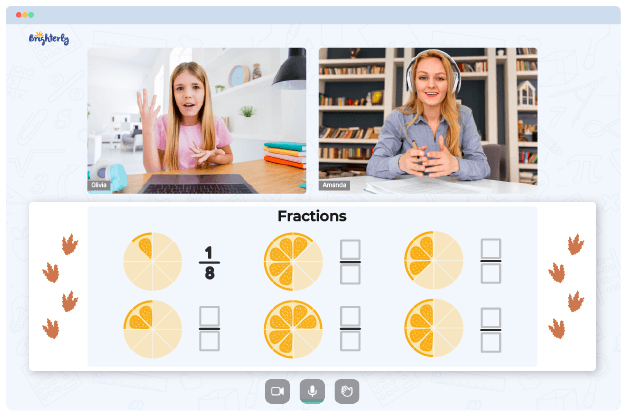# Decompose Fractions

Welcome to Brighterly, where learning math is made fun and engaging for children! In this article, we will delve into the fascinating world of fractions and learn how to decompose them. Understanding how to decompose fractions is a crucial skill that will strengthen your child’s grasp of fractional concepts and enhance their problem-solving abilities. So let’s jump right in and explore the wonders of decomposing fractions!

## What Is Decomposing Fractions?

Decomposing fractions is the process of breaking down a given fraction into smaller, more manageable parts. By decomposing fractions, we can express them as a sum of simpler fractions. This technique allows us to gain a deeper understanding of fractions and work with them more flexibly.

## How to Decompose Fractions?

To decompose fractions effectively, we can employ different strategies depending on the type of fraction. Let’s explore two main scenarios: decomposing fractions into unit fractions and decomposing fractions into non-unit fractions.

## How to Decompose Fractions into Unit Fractions?

Decomposing fractions into unit fractions involves expressing a fraction as a sum of fractions where the numerator is 1. For instance, if we have the fraction 3/4, we can decompose it as 1/4 + 1/4 + 1/4. By breaking down the original fraction into unit fractions, we gain insight into its constituent parts.

## How to Decompose Fractions into Non-Unit Fractions?

Decomposing fractions into non-unit fractions entails expressing a fraction as a sum of fractions where the numerator is greater than 1. For example, if we have the fraction 5/6, we can decompose it as 1/6 + 1/6 + 1/6 + 1/6 + 1/6. By decomposing the fraction in this manner, we can visualize its composition and work with it more effectively.

## How to Decompose Mixed Numbers?

When it comes to decomposing mixed numbers, we deal with fractions that have a whole number component. To decompose mixed numbers, we first convert them to improper fractions and then apply the techniques mentioned earlier. Decomposing mixed numbers enables us to understand the relationship between the whole and fractional parts more clearly.

## Solved Examples on Decompose Fractions

To solidify our understanding of decomposing fractions, let’s solve a few examples together:

1. Decompose the fraction 2/3 into unit fractions.
2. Decompose the fraction 7/8 into non-unit fractions.
3. Decompose the mixed number 3 1/2 into a sum of fractions.

By going through these solved examples step by step, your child will gain confidence in decomposing fractions and feel ready to tackle more complex problems.

## Practice Problems on Decompose Fractions

To further strengthen your child’s skills, we have prepared a set of practice problems on decomposing fractions. These exercises will allow your child to apply the techniques learned and reinforce their understanding of the topic. Practice makes perfect, so encourage your child to take on these challenges and excel in their fraction decomposition abilities!

## Conclusion

Congratulations! You have explored the captivating world of decomposing fractions with Brighterly. By understanding how to break down fractions into smaller components, your child has unlocked the power to manipulate fractions with ease. Decomposing fractions not only enhances their mathematical skills but also cultivates critical thinking and problem-solving abilities, setting them up for success in their academic journey. So keep practicing and let the vibrant world of fractions unfold before you with Brighterly.

## Frequently Asked Questions on Decompose Fractions

### Why is decomposing fractions important?

Decomposing fractions is important because it allows us to understand the composition of fractions more deeply. By breaking down fractions into smaller parts, we gain insights into their structure, which makes them easier to work with and manipulate. Decomposing fractions enhances our ability to solve complex problems involving fractions and strengthens our overall understanding of mathematical concepts.

### Are there other methods to decompose fractions?

Yes, apart from the strategies mentioned earlier, there are additional methods to decompose fractions. One such method is finding common denominators. By finding a common denominator for two or more fractions, we can express them with the same denominator, making it easier to decompose and perform operations on them. Another approach is finding equivalent fractions, which involves multiplying or dividing the numerator and denominator of a fraction by the same number to create an equivalent fraction with desired properties.

### How can I help my child grasp the concept of decomposing fractions?

Supporting your child in understanding the concept of decomposing fractions is crucial for their learning journey. Here are a few tips to help them grasp the concept:

1. Encourage visualization: Use visual aids such as fraction bars, manipulatives, or drawings to help your child visualize fractions and their decomposition. This hands-on approach helps them develop a concrete understanding of the concept.

2. Provide ample practice: Offer your child various practice opportunities with decomposing fractions. This can include solving practice problems, playing math games, or engaging in real-life scenarios where fractions are involved. Practice reinforces learning and builds confidence.

3. Foster exploration: Encourage your child to explore different strategies for decomposing fractions. Allow them to experiment with various decomposition methods and discuss their reasoning behind each approach. This promotes critical thinking and deepens their understanding.

4. Make it relatable: Connect the concept of decomposing fractions to real-life situations or practical examples. Show how fractions are used in cooking, measurements, or dividing objects among friends. Relating fractions to everyday experiences helps your child see the relevance and application of the concept.

Sources:

Troubles with Fractions?• Does your child struggle with mastering the concept of fractions?
• Try learning with an online tutor.

Is your child having difficulties with grasping fractions concepts? An online tutor could provide the necessary help.